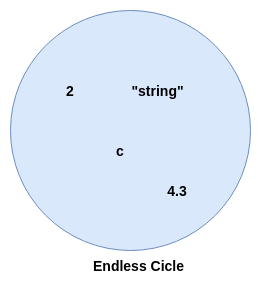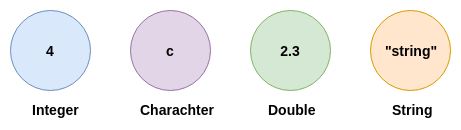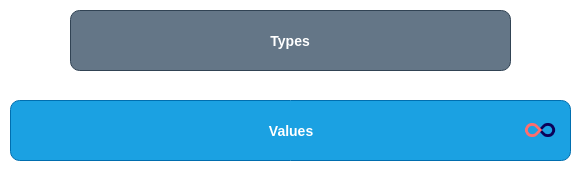Chapter 1: What are the types?
Before moving to the types, first, let see what are the values. Values are like raw material or anything: `2, c, “string”, 4.3,` and more. In mathematical terms, we can say values are infinite. Like in the diagram.We have an endless circle which contains the infinite number of elements or values. Those values are anything. For recognizing those values, types come into the picture. As we know, all computer science concepts are derived from mathematics, for recognizing the values from the endless circle, based on mathematics set-theory, simple type theory comes into the picture. These theories are a further huge topic, we are not exploring those in this book. Let's break this endless circle into a small set of circles based on their associated types.As you can see, now we have a separate set of values which are defined on the basis of their types, but still, the range of the set is based on the type only. Like integer set contains values on the basis of integer range, but it only contains integer type values.
Daniel Spiewak explains this in this one of his talk High Wizardry in the Land of Scala. In this, he explains the scope of the types and values like in this diagram:In this diagram, values are infinite, but types are less than values. Let's take an example of Scala, which explains.
1
val a: Int = 4
2
val b: Int = 5
3
4
val string: String = “Hello World”
Copied!
In this example, we have 3 values and two types and we know, with single type integer we can create value from -2147483648 to 2147483647 range. Same with the string type, we can create an infinite number of values, but the type is still the same which is called String. So, that’s why we can say that types are less than values.
After this whole conclusion, we can say that type is something similar to a specific type of container which holds the value on the basis of its properties and shape like for carrying the normal water we requires containers like glass, bottle, bucket and more but if we want to carry some chemicals, we requires a special safe container. So, on the basis of the values we require typing.